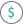#DDB (PQL - xl)

This function calculates the depreciation of an asset for a specified period using the double-declining balance method or another method you specify

• Library: PQL \ Spreadsheet \ Financial
• Compatibility: Any content (regardless of data source) in the Tabulate spreadsheet module

#### Syntax

DDB(cost, salvage, life, period, OPTIONAL factor)

##### Function Arguments
 Name Description Type Optional cost Initial cost of the asset Number salvage Value at the end of depreciation Number life Number of periods over which the asset is being depreciated Number period Period for which you want to calculate the depreciation in the same units as the life argument Number factor Rate at which the value declines; if omitted, the calculation uses 2 (double-declining method) Number Y